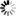Normal view

# Understanding political science statistics : observations and expectations in political analysis / Peter Galderisi.

Material type:TextLanguage: İngilizce Publisher: New York ; Milton Park, Abingdon, Oxon : Routledge, 2015Copyright date: ©2015Description: xxiii, 339 pages : charts, tables ; 24 cmContent type: text Media type: unmediated Carrier type: volumeISBN: 9780415890052LOC classification: JA71.7 | .G35 2015Other classification: POL000000
Contents:
Political science, the scientific method, and statistical analysis: An overview -- How do we measure and observe? -- Central tendency as summary observation -- Dispersion/Variation/Goodness of fit as summary observation -- Standardized scores and normal distributions: The concept of relative observation -- An intuitive introduction to inference and hypothesis testing -- Hypothesis testing and the concept of association: Observations and expectations about the difference between means -- Inferential statistics for proportions -- Measuring association for nominal and ordinal data -- Research design and the use of control variables -- Different by how much? Linear regression -- Retracing our methodological steps: Hypotheses, multiple regression, and the efffects of third variables.
Summary: "Any student can learn the basic concepts of statistics if they are introduced as solutions to particular problems, and not formulas with a life of their own. In this text, students are introduced to a problem, asked to consider, conceptually, how one would address that problem, and then led through the derivation of the appropriate statistical formula. This applied method of teaching statistics through political science examples allows students to see the research method as problem solving. They learn the math, but only after they learn the concepts and methodological considerations that give the math context. The concepts throughout are presented through the lens of "observations and expectations," applied to myriad statistical techniques, both descriptive and inferential, as well as more generalized concepts of research methodology itself, such as hypothesis testing. Galderisi highlights that with each advance in technical sophistication, each statistical procedure is built on a small set of basic concepts, such as the reasons for standardization, the effects of outliers, or the concept of proportional reduction of error, to show that they are cumulative. More important than just memorizing a series of formula, this text emphasizes the underlying logic of statistical analysis for greater understanding. Further, the applications and examples drawn from political science (including law) allow students to better see how they can apply these concepts and techniques in their own research and in future coursework"-- Provided by publisher.
Tags from this library: No tags from this library for this title.Average rating: 0.0 (0 votes)
Item type Current location Collection Call number Copy number Status Date due Barcode Course reserves
Reserve-1d Merkez Kütüphane
Ayrılmış Ders Kitapları / Course Reserves
Ayrılmış ders kitapları RES JA71.7 .G35 2015 (Browse shelf) 1 Available 0060090
Reserve-1d Merkez Kütüphane
Ayrılmış Ders Kitapları / Course Reserves
Ayrılmış ders kitapları RES JA71.7 .G35 2015 c.2 (Browse shelf) 2 Available 0060091

Includes Glossary.

Includes bibliographical references and index.

Political science, the scientific method, and statistical analysis: An overview -- How do we measure and observe? -- Central tendency as summary observation -- Dispersion/Variation/Goodness of fit as summary observation -- Standardized scores and normal distributions: The concept of relative observation -- An intuitive introduction to inference and hypothesis testing -- Hypothesis testing and the concept of association: Observations and expectations about the difference between means -- Inferential statistics for proportions -- Measuring association for nominal and ordinal data -- Research design and the use of control variables -- Different by how much? Linear regression -- Retracing our methodological steps: Hypotheses, multiple regression, and the efffects of third variables.

"Any student can learn the basic concepts of statistics if they are introduced as solutions to particular problems, and not formulas with a life of their own. In this text, students are introduced to a problem, asked to consider, conceptually, how one would address that problem, and then led through the derivation of the appropriate statistical formula. This applied method of teaching statistics through political science examples allows students to see the research method as problem solving. They learn the math, but only after they learn the concepts and methodological considerations that give the math context. The concepts throughout are presented through the lens of "observations and expectations," applied to myriad statistical techniques, both descriptive and inferential, as well as more generalized concepts of research methodology itself, such as hypothesis testing. Galderisi highlights that with each advance in technical sophistication, each statistical procedure is built on a small set of basic concepts, such as the reasons for standardization, the effects of outliers, or the concept of proportional reduction of error, to show that they are cumulative. More important than just memorizing a series of formula, this text emphasizes the underlying logic of statistical analysis for greater understanding. Further, the applications and examples drawn from political science (including law) allow students to better see how they can apply these concepts and techniques in their own research and in future coursework"-- Provided by publisher.

Share